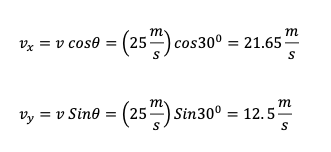# Practice – Solve problems involving 2D motion

Questions :

A racecar went off track at an angle of 30 degree. if the velocity of the car was 25m/s  at that moment, what was the x and y component of velocity?

A dolphin leaps out of the water at an angle of 35º above the horizontal. The horizontal component of the dolphin’s velocity is 7.7 m/s. Find the magnitude of the vertical component of the velocity.

There are 2 engines in a spacecraft. Engine A, accelerates the plane in the x producing acceleration of 5.10 m/s². Engine B accelerates the plane in y-direction producing an acceleration of 7.30 m/s². The engines fire up for 565 s. At the end of the firing period, the craft has velocity components of vx = 3775 m/s and vy = 4816 m/s. Find the magnitude and direction of the initial velocity of the plane.

Explanations :We can relate the launch angle and the velocity component with the trigonometry function.There are 2 engines in a spacecraft. Engine A, accelerates the plane in the x producing acceleration of 5.10 m/s². Engine B accelerates the plane in y-direction producing an acceleration of 7.30 m/s². The engines fire up for 565 s. At the end of the firing period, the craft has velocity components of vx = 3775 m/s and vy = 4816 m/s. Find the magnitude and direction of the initial velocity of the plane.

Step 1: What we knowStep 2 : What we need to know?

To know the initial velocity, we need to know the initial x and y component of velocity. So we need to know Vox and Voy. Once we know both the components, we can apply the Pythagorean theorem and Trigonometric functions to find the velocity and direction.Step 4: Calculate magnitude and direction of initial velocityScroll to Top